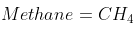## Combustion Processes

Methane burns in 30% excess air. What is the combustion equation?Hint
The stoichiometric combustion of methane in oxygen:
$$CH_4+2\:O_2\rightarrow CO_2+2\:H_2O$$$Hint 2 For combustion in air, there will be 3.76 moles of nitrogen for each mole of oxygen. Thus, the stoichiometric combustion of methane in air is: $$CH_4+2\:O_2+2(3.76)\:N_2\rightarrow CO_2+2\:H_2O+2(3.76)\:N_2$$$
The stoichiometric combustion of methane in oxygen:
$$CH_4+2\:O_2\rightarrow CO_2+2\:H_2O$$$For combustion in air, there will be 3.76 moles of nitrogen for each mole of oxygen. Thus, the stoichiometric combustion of methane in air is: $$CH_4+2\:O_2+2(3.76)\:N_2\rightarrow CO_2+2\:H_2O+2(3.76)\:N_2$$$
Because the problem statement is asking for combustion in excess air, the excess oxygen appears as oxygen on the right side of the combustion equation. In 30% excess air, multiply the air components on the reactants side by 1.3. This creates an excess of 0.6 ( $$2\:O_2 \times 1.3=2.6\:O_2\rightarrow 2.6\:O_2-2\:O_2=0.6\:O_2$$ ) units of air to add on the products side for balancing. Thus, the stoichiometric combustion of methane in excess air is:
$$CH_4+2.6\:O_2+2.6(3.76)\:N_2\rightarrow CO_2+2\:H_2O+2.6(3.76)\:N_2+0.6\:O_2$$$$$CH_4+2.6\:O_2+2.6(3.76)\:N_2\rightarrow CO_2+2\:H_2O+2.6(3.76)\:N_2+0.6\:O_2$$$